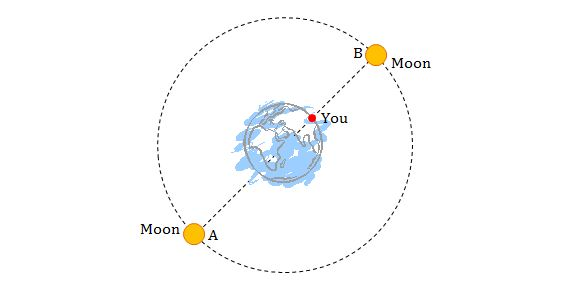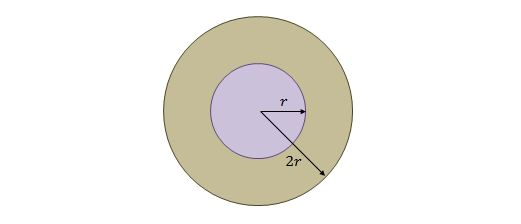Classical Mechanics

# Newton's Laws of Gravity

Climbing to the top of Mount Everest is hard, but it's slightly easier than you might think as people weigh less as they climb to the top. Let $$W_E$$ be a person's weight on top of Mount Everest and $$W_S$$ be their weight at sea level. What is the value of $$1-W_E/W_S$$?

Details and assumptions

• Assume the earth (other than Everest) is a sphere of mass $$6 \times 10^{24}~\mbox{kg}$$ and radius $$6,370~\mbox{km}$$.
• The top of Mount Everest is $$8,848~\mbox{m}$$ above the surface of the earth.If the moon moves from the opposite side of the earth from you (A in the above figure) to the facing side (B in the above figure), approximately by what percent does the moon's gravitational force on you (with mass $$65 \text{ kg}$$) increase, assuming that the earth-moon (center-to-center) distance is $$3.55 \times 10^8\text{ m}$$ and the earth's radius is $$6.37 \times 10^6\text{ m}?$$

Assume that the earth and the moon are both perfect spheres and the earth-moon distance is constant.

If the magnitude of gravitational attraction force between two particles with respective masses $$5.5\text{ kg}$$ and $$2.9\text{ kg}$$ is $$2.7 \times 10^{-12}\text{ N},$$ what is the approximate separation between the two particles, given that the universal gravitational constant is $$G=6.67 \times 10^{-11} \text{ N}\cdot\text{m}^2\text{/kg}^2?$$

A neutron star has a mass equal to that of the sun, which is $$2.1 \times 10^{30}\text{ kg},$$ but has a radius of only $$7\text{ km}.$$ What is the approximate gravitational acceleration at the surface of the star?

Assumptions and details

• The universal gravitational constant is $$G=6.67 \times 10^{-11} \text{ N}\cdot\text{m}^2\text{/kg}^2.$$The interior structure of the Earth is layered in spherical shells, like an onion. Assume that a certain planet has similar interior structure of core and outer shell, as shown in the above figure. The radius of the core is $$R$$ and the inner and outer radii of the outer shell are $$R$$ and $$2R,$$ respectively. If the mass of the core is $$M=4.1 \times 10^{24}\text{ kg}$$ and that of the outer shell is $$4M,$$ what is the approximate gravitational acceleration of a particle which is at a distance of $$R$$ from the surface of the outer shell?

• The universal gravitational constant is $$G=6.67 \times 10^{-11} \text{ N }\cdot\text{m}^2\text{/kg}^2.$$
• $$R = 5.5\times10^6$$ m.
×

Problem Loading...

Note Loading...

Set Loading...